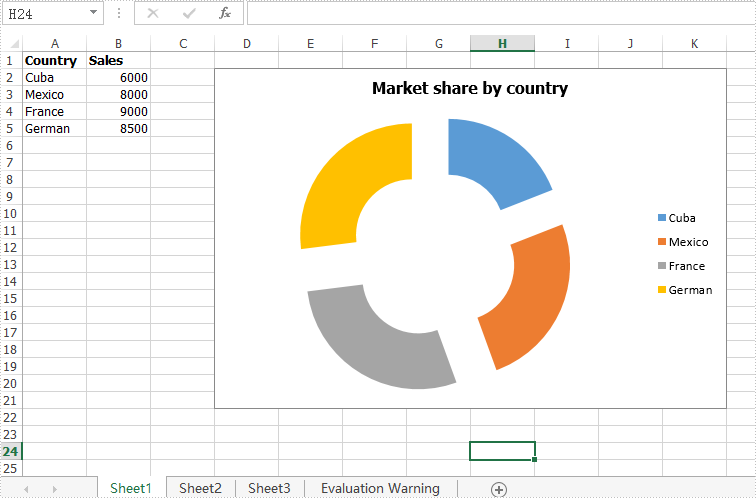# How to explode a doughnut chart in C#

In the previous article, we have demonstrated how to explode a pie chart via Spire.XLS. This article we will explain how to explode a doughnut chart in C#.

When we need to explode a doughnut chart, we can set the chart type as DoughnutExploded when we create a doughnut chart from scratch. For the existing doughnut chart, we can easily change the chart type to explode the doughnut chart.

Step 1: Create an instance of Excel workbook and load the document from file.

```Workbook workbook = new Workbook();
```

Step 2: Get the first worksheet from the sample workbook.

```Worksheet sheet = workbook.Worksheets;
```

Step 3: Get the first chart from the first worksheet.

```Chart chart = sheet.Charts;
```

Step 4: Set the chart type as DoughnutExploded to explode the doughnut chart.

```chart.ChartType = ExcelChartType.DoughnutExploded;
```

Step 5: Save the document to file.

```workbook.SaveToFile("ExplodedDoughnutChart.xlsx", ExcelVersion.Version2010);
```

Effective screenshot of the explode doughnut chart:Full codes of how to explode a doughnut chart:

```using Spire.Xls;
namespace ExplodeDoughnut
{

class Program
{

static void Main(string[] args)
{
Workbook workbook = new Workbook();